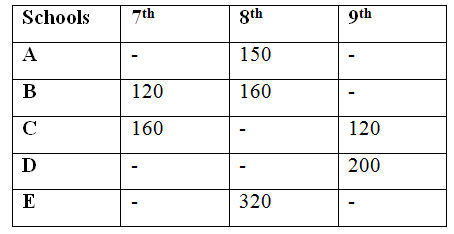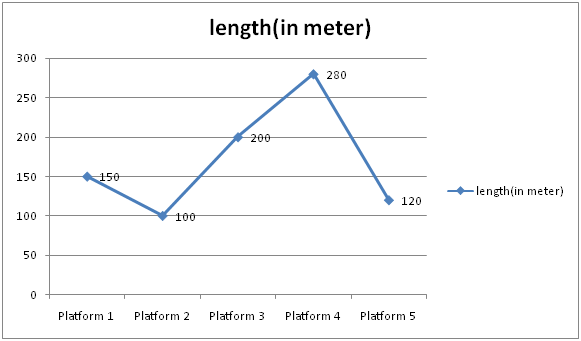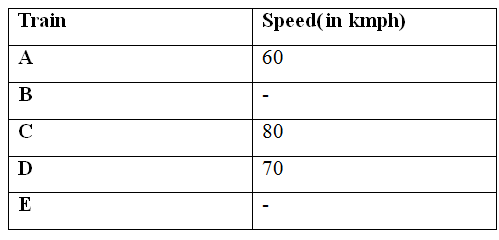# IBPS Clerk Mains Quantitative Aptitude Questions 2019 (Day-17) High Level New Pattern

Dear Aspirants, Quantitative Aptitude plays a crucial role in Banking and all other competitive exams. To enrich your preparation, here we have provided New Pattern Aptitude Questions for IBPS Clerk Mains. Candidates those who are going to appear in IBPS Clerk Mains can practice these questions daily and make your preparation effective.

[WpProQuiz 4892]

Directions (1-5): Study the following information carefully and answer the given questions:

The given table shows the number of students in three classes namely7th, 8th and 9th at five different schools. Some of the data are missing in the table.1) If the total number of students in the class 8th of all the school is 900 and the total number of students in school C and D is 400 and 470 respectively. Find the total number of students in class 7 of school D?

a) 120

b) 140

c) 150

d) 100

e) None of these

2) If the number of students in class 9th of school A is 10 more than the number of students in class 9th of school B respectively. The total number of students of school B and school A is 400 and 420. The ratio of the number of students in class 7th of school D and A is 5:7 and the average number of students in school E is 180. If the ratio of the number of students of class 9 and class 7 of school E is 6:5, then what is the average number of students in class 7th of all the five schools?

a) 124

b) 126

c) 128

d) 122

e) None of these

3) If the number of students in class 9th of school B is twice the number of students in class 9th of school A and the number of students in class 9 of school E is 20 more than the number of students in that same class of school A. The number of students of class 8 of school C is 105(5/19)% of the number of students of class 9 of school E. The average number of students in class 9 of all five schools is 128, then what is the ratio of the total number students in school B to the total number of students of school C?

a) 43:38

b) 41:37

c) 45:32

d) Cannot be determined

e) None of these

4) The total number of students of school A and D is 400 and 420 respectively. The ratio of the number of students in class 9th of school A to the number of students in class 8th of school C is 2:3. The average number of students in class 7th of all the five schools together is 130 and number of class 8th students of school D is 20% more than that of the number of students in class 7th of school D. If the ratio of the number of students of class 7th of school E and A is 5:4, what is the total number of students in class 8th of all school?

a) 850

b) 800

c) 1200

d) 1000

e) 900

5) The number of students of class 8th D is 60 more than that of the number of students of class 8th in school C. The number of students in class 7 of school A and D is 20% and 80% more than the number of students in class 7 of school E respectively. The number of students of class 8th of school C is 80% of the number of class 8th of school A. The average number of students in class 8th of all the five schools is 46 more than the average number of students in class 7 of all the five schools, then what is sum of the number of students in class 7th of school D and A together?

a) 215

b) 320

c) 315

d) 325

e) None of these

Directions (6-10): Study the following information carefully and answer the given questions:

The given line graph shows the length of the platform (in meter).The given table shows the speed of the train.6) What is the length of train B?

Statement I: The speed of a train B is 50% more than that of the speed of train A and Train A will cross the platform4 in 36 seconds.

Statement II: Train A and train B running in the opposite direction and the train A crosses the train B in 18 seconds.

a) Only I

b) Only II

c) Either I or II are sufficient

d) All I and II necessary to the answer the question

e) The question can’t be answered even with all I and II

7) The Length of train E is 300m and it takes 18 seconds to cross the platform5 completely and the train C crosses the platform3 in 36 seconds. If Train E and train C running in same direction, in what time will they be clear of each other from the moment they meet?

a) 410 seconds

b) 810 seconds

c) 840 seconds

d) 800 seconds

e) None of these

8)

Quantity I: Two trains D and C are of equal length and running in same direction. The faster train passes the slower train in 54 seconds. The length of each train is?

Quantity II: Train B crosses the platform5 in 54 seconds and the length of train B is 800% of the length of the platform. What is the speed of the train?

a) Quantity I > Quantity II

b) Quantity I ≥ Quantity II

c) Quantity II > Quantity I

d) Quantity II ≥ Quantity I

e) Quantity I = Quantity II or Relation cannot be established

9) Train B overtakes two persons who are walking in the same direction as the train is moving at speed of 3kmph and 6kmph respectively in 6seconds and 9 seconds respectively. Then the train B crosses the platform2 in x seconds. Find the value of x?

a) 34.5 seconds

b) 27.5 seconds

c) Cannot be determined

d) 31.5 seconds

e) None of these

10) The ratio of the length of train C and train B is 5:4. Train B cross the pole in 12 seconds and the train C cross the platform1 in 18 seconds. If train B and train E running opposite direction, cross each other in 12 seconds, and length of the train E is 120% of the length of train C, then find the speed of train E?

a) 150kmph

b) 120kmph

c) 180kmph

d) 100kmph

e) None of these

Direction (1-5) :

Number of students in class 8 of school C=400-(160+120) =120

Number of students in class 8 of school D=900-(150+160+120+320) =150

Number of students in class 7 of school D=470-(150+200) =120

Number of students of class 9th of school B=400-(120+160) =120

Number of students of class 9th of school A=120+10=130

Number of students of class 7th of school A=420-(150+130)=140

Number of students of class 7th of school D=5/7*140=100

Total number of students of school E=180*3=540

Total number of students of class 7th and class 9th of school E=540-320=220

Number of students of class 7th of school E=5/11*220=100

Required average= (140+120+160+100+100)/5=124

Number of students of class 9 of school A=x

Number of students of class 9 of school B=2x

Number of students of class 9 of school E=x+20

(x+2x+x+20+120+200)/5=128

x=75

Number of students of class 9 of school B=2(75) =150

Total Number of students of school B=120+160+150=430

Number of students of class 9 of school E=75+20=95

Number of students of class 8 of school C=105(5/19)% *95=100

Total Number of students of school C=160+100+120=380

Required ratio=430:380=43:38

Number of students in school D=420

Let Number of students of class 7th of school D=x

Number of students of class 8th of school D=x*120/100=6x/5

200+x+6x/5=420

11x/5=220

x=100

Number of students of class 7th of school D=100

Number of students of class 8th of school D=600/5=120

Total number of students of class 7 of all the 5 schools together=130*5=650

Total Number of class 7 students of school E and A=650-(120+160+100)=270

Number of students in Class 7 of school A=5/9*270=150

Number of students in Class 9 of school A=400-(150+150)=100

Number of students in Class 8th of school C=3/2*100=150

Required total=150+160+150+120+320=900

Number of students of class 8th of school C=80/100*150=120

Number of students of class 8th of school D=120+60=180

Average number of students of class 8th in all the five schools

= (150+160+120+180+320)/5

=186

Average number of students of class 7th in all the five schools=186-46=140

Total number of students of class 7th of all the five schools=140*5=700

Let Number of students of class 7th of school E=x

Number of students of class 7th of school A=x*120/100=6x/5

Number of students of class 7th of school D=x*180/100=9x/5

(x+6x/5+9x/5+120+160)=700

20x/5=420

x=105

Thus Number of students of class 7th of school A=6*105/5=126

Number of students of class 7th of school D=9*105/5=189

Required sum=126+189=315

Direction (6-10) :

From Statement I,

Speed of train B=150/100*60=90kmph

Length of train A=X

X+280=60*5/18*36

X=600-280=320m

So, Statement I alone is not sufficient to the answer the question.

From Statement II,

Train A and train B running in the opposite direction and the train A crosses the train B in 18 seconds.

So, Statement II alone is not sufficient to the answer the question.

From I and II,

Length of train B=y

320+y=(90+60)*5/18*18

y=750-320=430m

Speed of train E*5/18=(300+120)/18

Speed train E=84kmph

Length of train C+200=80*5/18*36

Length of train C=600m

Time= (300+600)/((84-80)*5/18)

Time=900*18/(4*5)=810 seconds

From Quantity I,

Length of the train D=x

(x+x)=(80-70)*5/18*54

2x=10*5*3

x=75m

From quantity II,

Length of train B=120*800/100=960m

(960+120)=(speed of train)*5/18*54

1080=speed of train*15

Speed of train=72kmph

Length of train B=x

Speed of the train B=y

x=(y-3)*5/18*6

3x=5y-15

3x+15=5y

x=(y-6)*5/18*9

2x=5y-30

2x+30=5y

2x+30=3x+15

x=15m

Length of the train x=15m

5y=3(15) +15

5y=60

y=12kmph

Required time x=(15+100)/(12*5/18)

x=115*18/12*5=34.5 seconds

Length of train C =x

x+150=80*5/18*18

x=400-150=250m

Length of train B=4/5*250=200m

Length of train E=120/100*250=300m

Speed of train E*5/18=500/12=1800/12=150kmph